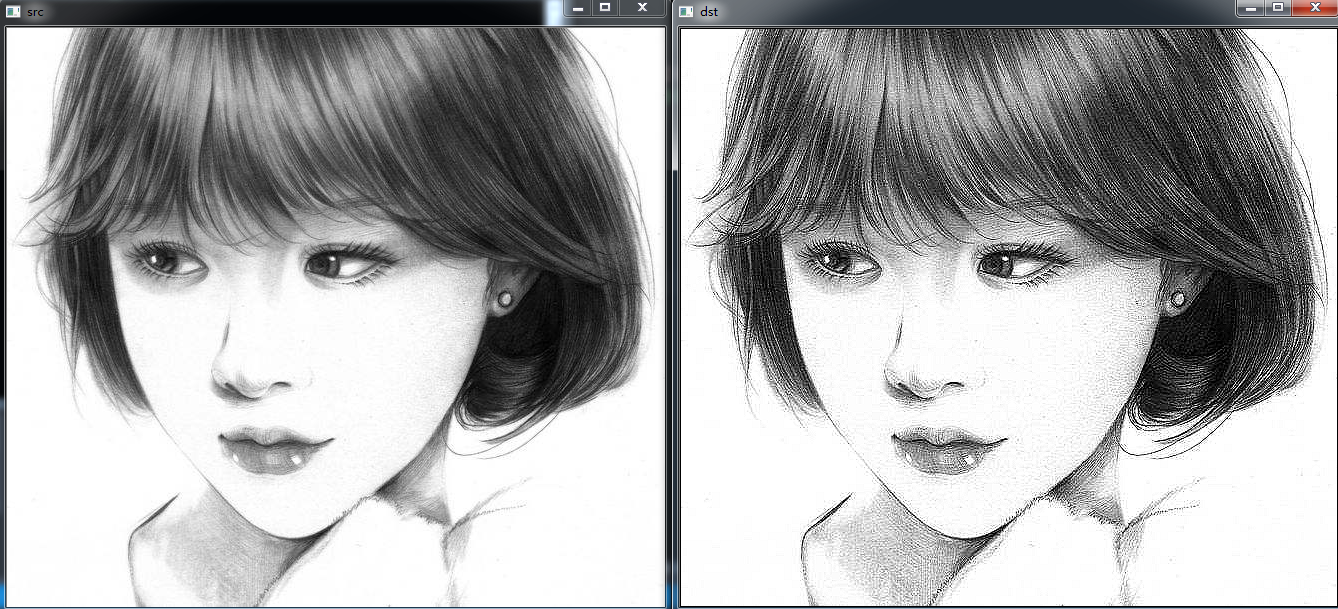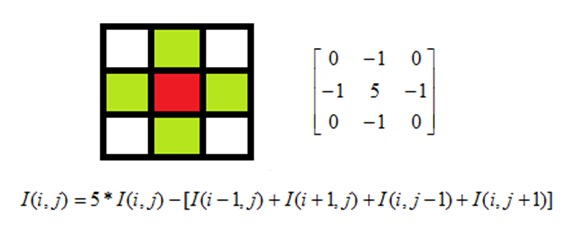2016-12-05 17:27:18 jinxiaonian11 阅读数 67042
• ###### MATLAB图像处理

全面系统的学习MATLAB在图像处理中的应用

19908 人正在学习 去看看 魏伟

①提取感兴趣区,用预先制作的感兴趣区掩模与待处理图像相乘,得到感兴趣区图像,感兴趣区内图像值保持不变,而区外图像值都为0。
②屏蔽作用,用掩模对图像上某些区域作屏蔽,使其不参加处理或不参加处理参数的计算,或仅对屏蔽区作处理或统计。
③结构特征提取,用相似性变量或图像匹配方法检测和提取图像中与掩模相似的结构特征。
④特殊形状图像的制作。

2019-06-15 00:06:38 matt45m 阅读数 89
• ###### MATLAB图像处理

全面系统的学习MATLAB在图像处理中的应用

19908 人正在学习 去看看 魏伟

## 前言

1.数字图像处理中的掩膜的概念是起源于于PCB制版的过程，在半导体制造中，许多芯片工艺步骤采用光刻技术，用于这些步骤的图形“底片”称为掩膜（也称作“掩模”），其作用是：在硅片上选定的区域中对一个不透明的图形模板遮盖，继而下面的腐蚀或扩散将只影响选定的区域以外的区域。
2.图像掩膜与其类似，用选定的图像、图形或物体，对处理的图像（全部或局部）进行遮挡，来控制图像处理的区域或处理过程。

3.数字图像处理中,图像掩模主要用于：
①提取感兴趣区,用预先制作的感兴趣区掩模与待处理图像相乘,得到感兴趣区图像,感兴趣区内图像值保持不变,而区外图像值都为0。
②屏蔽作用,用掩模对图像上某些区域作屏蔽,使其不参加处理或不参加处理参数的计算,或仅对屏蔽区作处理或统计。
③结构特征提取,用相似性变量或图像匹配方法检测和提取图像中与掩模相似的结构特征。
④特殊形状图像的制作。
4.掩膜是一种图像滤镜的模板，实用掩膜经常处理的是遥感图像。当提取道路或者河流，或者房屋时，通过一个n*n的矩阵来对图像进行像素过滤，然后将我们需要的地物或者标志突出显示出来。这个矩阵就是一种掩膜。

## 代码演示

1.下面是用掩膜来增加图像对比度的函数，可以看它实现的公式：

**I(i,j)=5∗I(i,j)−[I(i−1,j)+I(i+1,j)+I(i,j−1)+I(i,j+1)]**

void mask(Mat &input, Mat &dest)
{
//生成一个和源图像大小相等类型相同的全0矩阵
dest = Mat::zeros(input.size(), input.type());
//获取图像的列数
int cols = (input.cols - 1)*input.channels();
//获取图像的行数
int rows = input.rows;
//获取图像通道数
int channel = input.channels();

//遍历行数
for (int row = 1; row < rows - 1; row++)
{
//上一行
uchar* previous = input.ptr<uchar>(row - 1);
//当前行
uchar* current = input.ptr<uchar>(row);
//下一行
uchar* next = input.ptr<uchar>(row + 1);

//输出图像的行
uchar* output = dest.ptr<uchar>(row);
//开始对每个像素做掩膜操作
for (int col = channel; col < cols; col++)
{
output[col] = saturate_cast<uchar>(5 * current[col] - (current[col - channel] + current[col + channel] + previous[col] + next[col]));
}
}
}2017-01-13 19:54:11 i_chaoren 阅读数 3304
• ###### MATLAB图像处理

全面系统的学习MATLAB在图像处理中的应用

19908 人正在学习 去看看 魏伟if (二值图掩膜区像素 == 255)  {

for(int k=0;k<3;k++)

原彩色图对应的区域 = 255;  }

if ( 二值图掩膜区像素== 255)

{ 使用9×9的模板对原图进行卷积滤波 }2019-01-04 00:25:59 qq_42887760 阅读数 376
• ###### MATLAB图像处理

全面系统的学习MATLAB在图像处理中的应用

19908 人正在学习 去看看 魏伟

# 1. 获取图像像素指针

• CV_Assert(myImage.depth() == CV_8U);
• Mat.ptr(int i=0) 获取像素矩阵的指针，索引i表示第几行，从0开始计行数。
• 获得当前行指针const uchar* current= myImage.ptr(row );
• 获取当前像素点P(row, col)的像素值 p(row, col) =current[col]

# 2. 像素范围处理saturate_cast

saturate_cast（-100），返回 0。
saturate_cast（288），返回255
saturate_cast（100），返回100

# 3. 掩膜操作实现图像对比度调整• 掩膜矩阵 3*3 在图像矩阵上移动与图像重合，与每一个重合的像素点做掩膜操作，

• 公式：中心点掩膜后的颜色数据 $I(i,j) = 5*I(i,j) - [I(i-1,j)+I(i+1,j)+I(i,j-1)+I(i,j+1)]$

• 这里是3*3的矩阵，所以图像数据的第一行倒数第一行，第一列倒数第一列不做掩膜操作 。其中： i，j 表示像素的位置，第 i 行，第 j 列， I（i，j） 表示每个通道颜色数据。

• 掩膜操作不是矩阵乘法，由公式可以看出

• 该掩膜矩阵的作用： 掩膜操作可以提高图像对比度，对比度提高可以增加图像感官度、锐化，让看起来有点模糊的图像更清晰

###### 程序代码
#include<opencv2/opencv.hpp>
#include<iostream>
#include<math.h>

using namespace cv;

int main(int argc,char** argv){
Mat src,dst;

if(!src.data){
return -1;
}
//CV_Assert(src.depth()==CV_8U);

//掩膜操作
int channels=src.channels();//图像的通道数
int cols=(src.cols)*src.channels();//列数*通道数
int rows=src.rows;//行数

dst=Mat::zeros(src.size(),src.type());//初始化 dst

for(int row=1 ; row<rows-1 ; row++){
const uchar* previous = src.ptr<uchar>(row - 1);//上一行
const uchar* current=src.ptr<uchar>(row);//当前行
const uchar* next =src.ptr<uchar>(row + 1);//下一行

uchar* output=dst.ptr<uchar>(row);
for(int col=1*channels ; col<cols-1*channels ; col++){
//掩膜操作：I(i,j) = 5*I(i,j) - [I(i-1,j)+I(i+1,j)+I(i,j-1)+I(i,j+1)]
output[col] =saturate_cast<uchar>( 5 * current[col] - (previous[col] + next[col] + current[col + channels] + current[col + channels]));
}

}

namedWindow("output1",CV_WINDOW_AUTOSIZE);
imshow("output1",src);

namedWindow("output2",CV_WINDOW_AUTOSIZE);
imshow("output2",dst);

waitKey(0);

return 0;
}

###### 运行效果（即：将32行中output[col] =saturate_cast( 5 * current[col] - (previous[col] + next[col] + current[col + channels] + current[col + channels]));# 4. 函数调用filter2D功能

• 定义掩膜：Mat kernel = (Mat_(3,3) << 0, -1, 0, -1, 5, -1, 0, -1, 0);
• filter2D( src, dst, src.depth(), kernel ); 其中src与dst是Mat类型变量、src.depth表示位图深度，有32、24、8等。
###### 代码
#include<opencv2/opencv.hpp>
#include<iostream>
#include<math.h>

using namespace cv;

int main(int argc,char** argv){
Mat src,dst;

if(!src.data){
return -1;
}

Mat kernel=(Mat_<char>(3,3)<< 0, -1, 0, -1, 5, -1 , 0, -1, 0);
filter2D(src,dst,src.depth(),kernel);
//filter2D(src,dst,-1,kernel);

namedWindow("output1",CV_WINDOW_AUTOSIZE);
imshow("output1",src);

namedWindow("output2",CV_WINDOW_AUTOSIZE);
imshow("output2",dst);

waitKey(0);

return 0;
}

###### 运行效果（与上述代码效果一致）：# 5.测试运行时间

......
#include <time.h>
......
int main(){
clock_t start, finish;
double  duration;

start = clock();

//......
//要执行的内容
//......

finish = clock();
duration = (double)(finish - start) / CLOCKS_PER_SEC;
printf("行优先用时： %f seconds\n", duration);

return 0;
}


......
......
int main(){
double t=getTickCount();

//......
//要执行的内容
//......

double timeConsume = (getTickCount() - t) / getTickFrequency();
printf("time consume %.2f",timeConsume);

return 0;
}

###### 案例代码：
#include<opencv2/opencv.hpp>
#include<iostream>
#include<math.h>

using namespace cv;

int main(int argc,char** argv){
Mat src,dst;

if(!src.data){
return -1;
}

double t=getTickCount();

Mat kernel=(Mat_<char>(3,3)<< 0, -1, 0, -1, 5, -1 , 0, -1, 0);
filter2D(src,dst,src.depth(),kernel);
//filter2D(src,dst,-1,kernel);

double timeConsume = (getTickCount() - t) / getTickFrequency();
printf("time consume %.2f",timeConsume);

namedWindow("output1",CV_WINDOW_AUTOSIZE);
imshow("output1",src);

namedWindow("output2",CV_WINDOW_AUTOSIZE);
imshow("output2",dst);

waitKey(0);

return 0;
}


2015-07-07 20:16:37 sinat_22822467 阅读数 1112
• ###### MATLAB图像处理

全面系统的学习MATLAB在图像处理中的应用

19908 人正在学习 去看看 魏伟